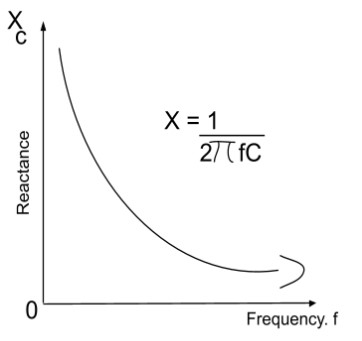# Capacitive Reactance Calculator

To find the capacitive reactance, enter the values of the frequency and the capacitance of the capacitor into the capacitive reactance calculator.

Formula:
Xc = 12π fC
Hz
F

Give Us Feedback

Capacitive reactance calculator can calculate the value of reactance of a capacitor using the frequency and the capacitance of capacitor.

## What is capacitive reactance?

“The resistance experienced by the flow of current between the walls of a capacitor is called capacitive reactance.”## Capacitive reactance formula

The equation used for capacitive reactance:

XC = 1/2πfC

In the above equation:

Xc refers to capacitive reactance,

f represents the frequency, and

C is capacitance.

## How to calculate capacitive reactance?

Example:

Find the capacitive reactance of a capacitor if the capacitance is 80 microfarads and the frequency is 200 hertz.

Solution

Step 1: Identify the values.

C = 80 F

f = 200 Hz

Step 2: Write the formula and put the given values in it.

Xc = 1/2πfC

Xc = 1/2π(200)(80)

Xc = 9.947 Ω

### Math Tools